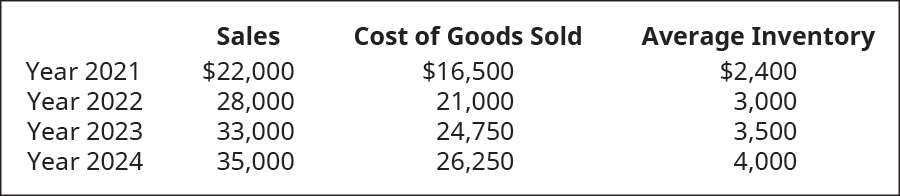# Use the following information relating to Shana Company to calculate the inventory turnover ratio and the number of days’ sales in inventory ratio.FindFindarrow_forward

### Principles of Accounting Volume 1

19th Edition
OpenStax
Publisher: OpenStax College
ISBN: 9781947172685

#### Solutions

Chapter
SectionFindFindarrow_forward

### Principles of Accounting Volume 1

19th Edition
OpenStax
Publisher: OpenStax College
ISBN: 9781947172685
Chapter 10, Problem 15PA
Textbook Problem
3 views

## Use the following information relating to Shana Company to calculate the inventory turnover ratio and the number of days’ sales in inventory ratio.To determine

Concept introduction:

Inventory Turnover Ratio:

Inventory Turnover Ratio measures the efficiency of the company in converting its inventory into sales. It is calculated by dividing the Cost of goods sold by Average inventory. The formula of the Inventory Turnover Ratio is as follows:

Inventory Turnover Ratio=Cost of goods soldAverage inventory

Note: Average inventory is calculated with the help of following formula:

Average inventory=(Beginning inventory + Ending inventory)2

Day’s sales in inventory:

Days sales in inventory represent the number of days the inventory waits for the sale. It is calculated using the following formula:

Day Sales in Inventory =  Inventory ×365Cost of Goods Sold

To calculate:

The Inventory Turnover Ratio and Number of Day’s sales in inventory ratio

### Explanation of Solution

The Inventory Turnover Ratio and Number of Day’s sales in inventory ratio are calculated as follows:

 Year 2021 Year 2022 Year 2023 Year 2024 Cost of Goods Sold (A) $16,500$ 21,000 $24,750$ 26,250 Average Inventory (B)

### Still sussing out bartleby?

Check out a sample textbook solution.

See a sample solution

#### The Solution to Your Study Problems

Bartleby provides explanations to thousands of textbook problems written by our experts, many with advanced degrees!

Get Started

Find more solutions based on key concepts
What is a biometric time clock?

Accounting Information Systems

The controller should be a member of the top management staff. Do you agree or disagree? Explain.

Managerial Accounting: The Cornerstone of Business Decision-Making

Differentiate price competition and non-price competition.

Foundations of Business (MindTap Course List)

How would each of the following changes tend to affect aggregate (i.e., the average for all corporations) payou...

Fundamentals of Financial Management, Concise Edition (with Thomson ONE - Business School Edition, 1 term (6 months) Printed Access Card) (MindTap Course List)